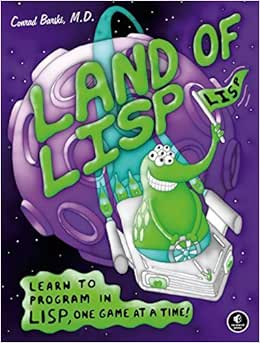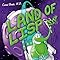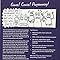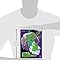# Land of Lisp: Learn to Program in Lisp, One Game at a Time! 平装

 亚马逊价格 全新品最低价 非全新品最低价
 Kindle电子书 "请重试" — —

•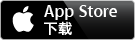•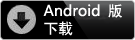Android手机或平板电脑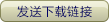## 基本信息

• 语言 ‏ : ‎ 英语
• ISBN ‏ : ‎ 1593272812
• 品牌 ‏ : ‎ No Starch Press,US
• 尺寸 ‏ : ‎ 17.93 x 3.1 x 23.5 cm
• 用户评分:

## 买家评论

5.0 颗星，最多 5 颗星
5星，共 5 星
7 买家评级
 4 星 0% (0%) 0% 3 星 0% (0%) 0% 2 星 0% (0%) 0% 1 星 0% (0%) 0%

### 7 个顾客评论

2016年6月3日(defun robots ()
(loop named main
with directions = '((q . -65) (w . -64) (e . -63) (a . -1)
(d . 1) (z . 63) (x . 64) (c . 65))
for pos = 544
then (progn (format t "~%qwe/asd/zxc to move, (t)eleport, (l)eave:")
(force-output)
(d (assoc c directions)))
(cond (d (+ pos (cdr d)))
((eq 't c) (random 1024))
((eq 'l c) (return-from main 'bye))
(t pos))))
for monsters = (loop repeat 10
collect (random 1024))
then (loop for mpos in monsters
collect (if (> (count mpos monsters) 1)
mpos
(cdar (sort (loop for (k . d) in directions
for new-mpos = (+ mpos d)
collect (cons (+ (abs (- (mod new-mpos 64)
(mod pos 64)))
(abs (- (ash new-mpos -6)
(ash pos -6))))
new-mpos))
'<
:key #'car))))
when (loop for mpos in monsters
always (> (count mpos monsters) 1))
return 'player-wins
do (format t
"~%|~{~<|~%|~,65:;~A~>~}|"
(loop for p
below 1024
collect (cond ((member p monsters)
(cond ((= p pos) (return-from main 'player-loses))
((> (count p monsters) 1) #\#)
(t #\A)))
((= p pos)
#\@)
(t
#\ ))))))

;; Loaded file c11-robots.lisp
T
> (robots)

| A |
| |
| A |
| A |
| A |
| |
| A |
| |
| A @ A |
| A |
| |
| |
| A |
| |
| A |
| |
qwe/asd/zxc to move, (t)eleport, (l)eave: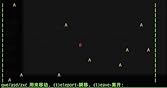6 个人发现此评论有用